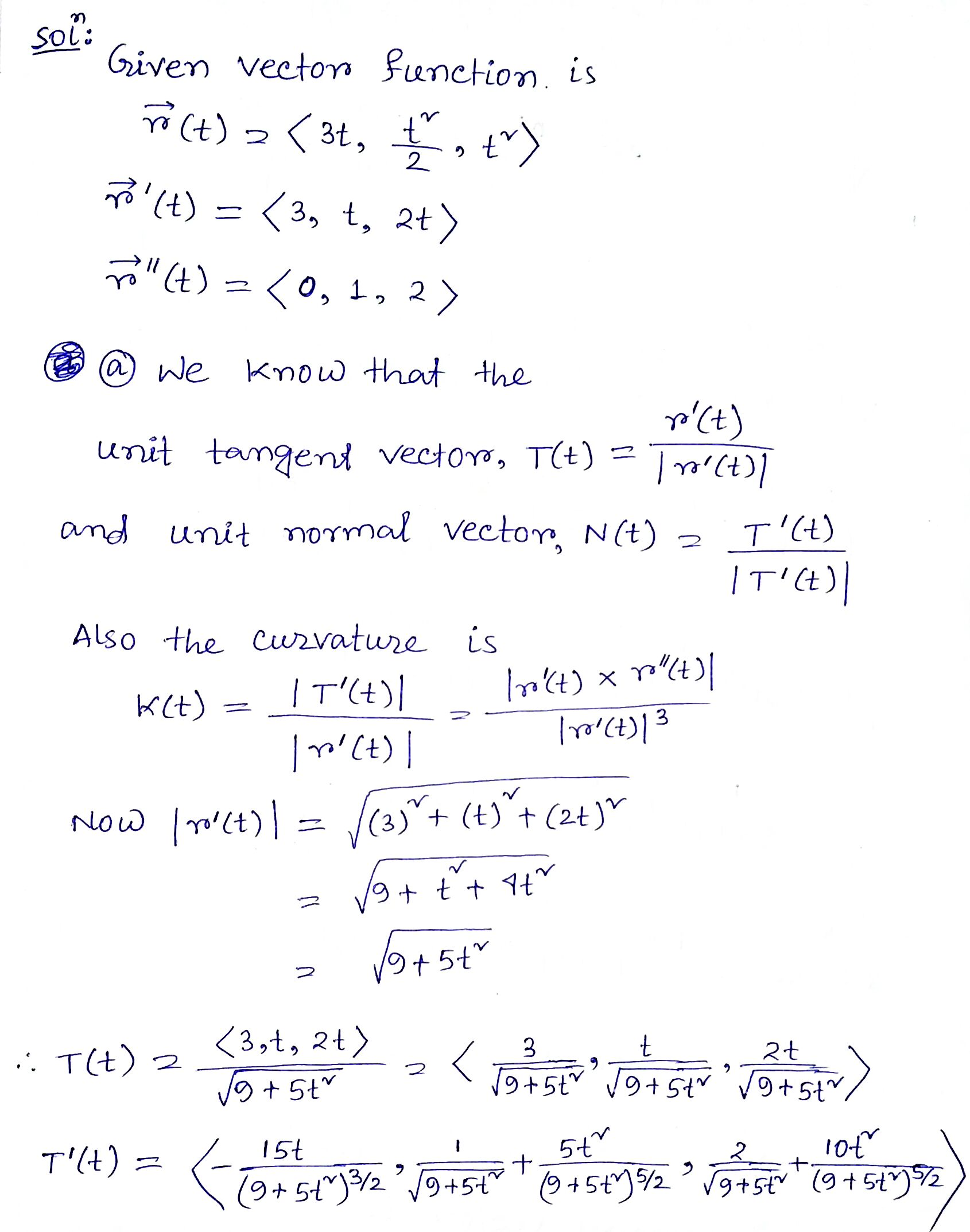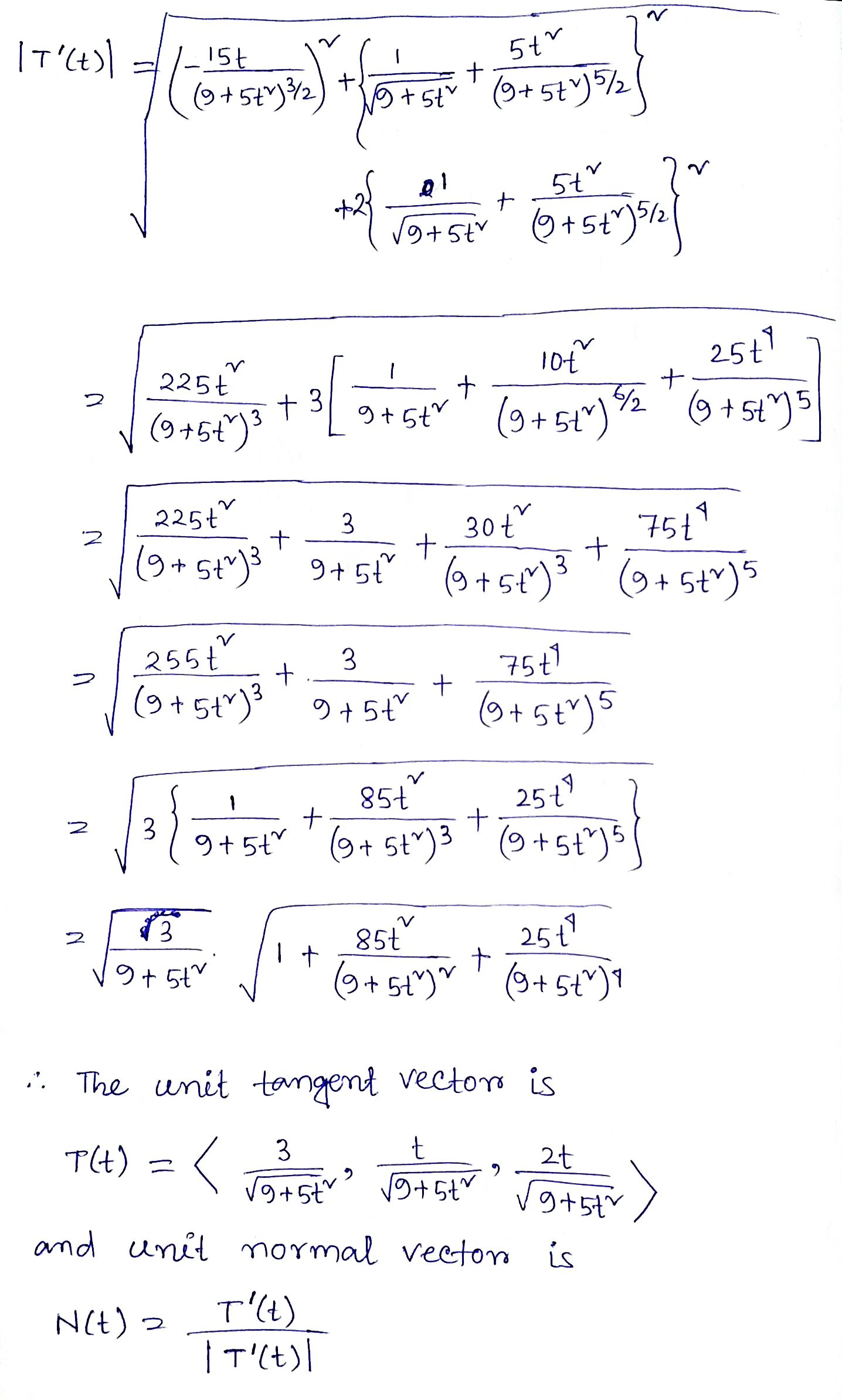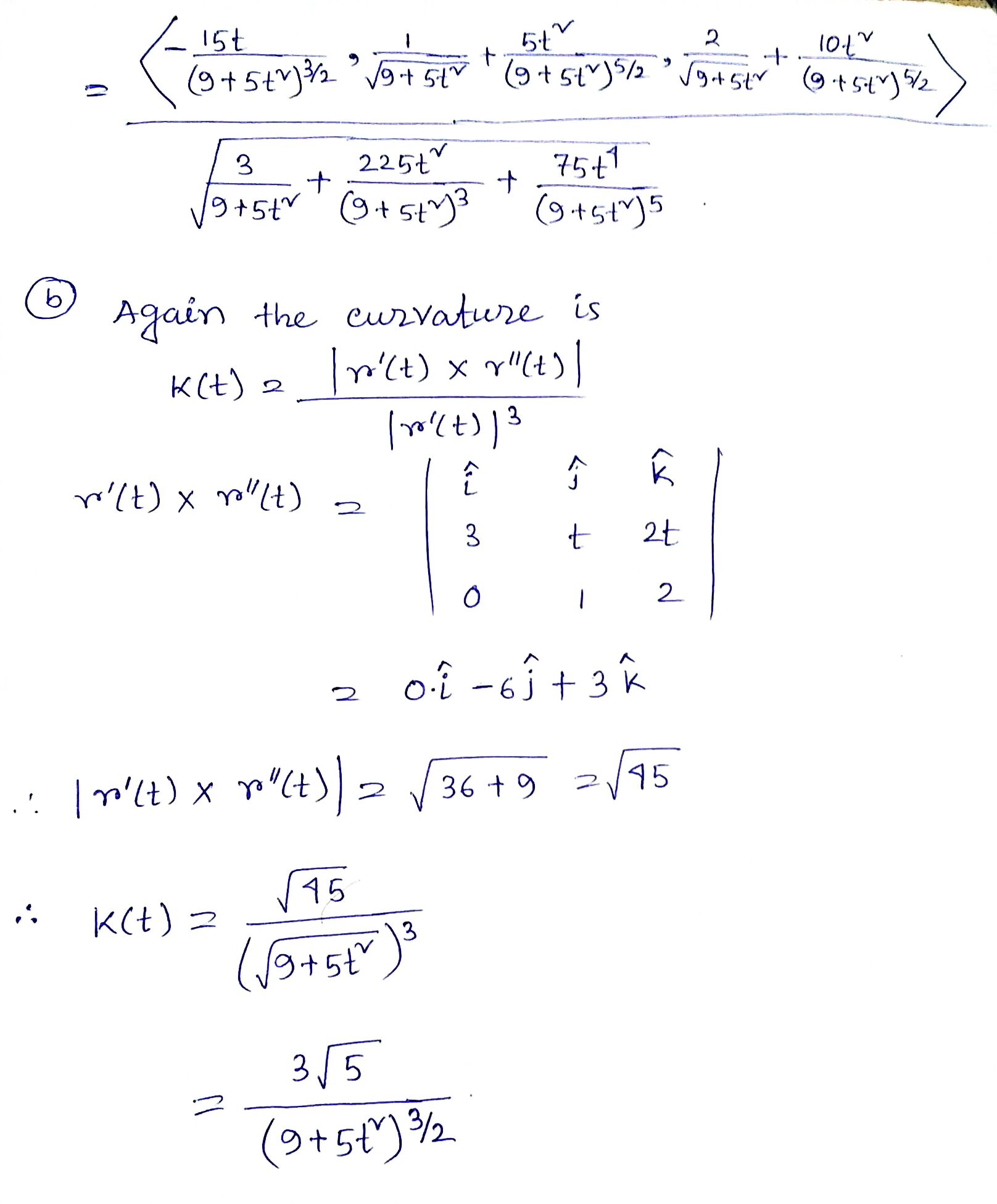In: Math

# Consider the following vector function. r(t) =<3t, 1/2 t2, t2> (a) Find the unit tangent and...

Consider the following vector function. r(t) =<3t, 1/2 t2, t2> (a) Find the unit tangent and unit normal vectors T(t) and N(t).

(b). Find the curvature k(t).

## Solutions

##### Expert Solution## Related Solutions

##### Consider the helix r(t)=(cos(2t),sin(2t),−3t)r(t)=(cos(2t),sin(2t),−3t). Compute, at t=π/6 A. The unit tangent vector T=T= ( , ,...
Consider the helix r(t)=(cos(2t),sin(2t),−3t)r(t)=(cos(2t),sin(2t),−3t). Compute, at t=π/6 A. The unit tangent vector T=T= ( , , ) B. The unit normal vector N=N= ( , , ) C. The unit binormal vector B=B= ( , , ) D. The curvature κ=κ=
##### 15. a. Find the unit tangent vector T(1) at time t=1 for the space curve r(t)=〈t3...
15. a. Find the unit tangent vector T(1) at time t=1 for the space curve r(t)=〈t3 +3t, t2 +1, 3t+4〉. b. Compute the length of the space curve r(t) = 〈sin t, t, cos t〉 with 0 ≤ t ≤ 6.
##### (1 point) For the given position vectors r(t)r(t) compute the unit tangent vector T(t)T(t) for the...
(1 point) For the given position vectors r(t)r(t) compute the unit tangent vector T(t)T(t) for the given value of tt . A) Let r(t)=〈cos5t,sin5t〉 Then T(π4)〈 B) Let r(t)=〈t^2,t^3〉 Then T(4)=〈 C) Let r(t)=e^(5t)i+e^(−4t)j+tk Then T(−5)=
##### compute the unit tangent vector T and the principal normal unit vector N of the space...
compute the unit tangent vector T and the principal normal unit vector N of the space curve R(t)=<2t, t^2, 1/3t^3> at the point when t=1. Then find its length over the domain [0,2]
##### Given the vector function r(t)=〈√t , 1/(t-1) ,e^2t 〉 a) Find: ∫ r(t)dt b) Calculate the...
Given the vector function r(t)=〈√t , 1/(t-1) ,e^2t 〉 a) Find: ∫ r(t)dt b) Calculate the definite integral of r(t) for 2 ≤ t ≤ 3 can you please provide a Matlab code?
##### Consider the vector function given below. r(t) = 2t, 3 cos(t), 3 sin(t) (a) Find the...
Consider the vector function given below. r(t) = 2t, 3 cos(t), 3 sin(t) (a) Find the unit tangent and unit normal vectors T(t) and N(t). T(t)   =    N(t)   =    (b) Use this formula to find the curvature. κ(t) =
##### Show the complete solution. Determine the unit tangent vector (T), the unit normal vector (N), and...
Show the complete solution. Determine the unit tangent vector (T), the unit normal vector (N), and the curvature of ?(?) = 2? ? + ?^2 ? – 1/3 ?^3 k at t = 1.
##### FOR THE PARAMETRIZED PATH r(t)= e^tcos(πt)i+e^tsin(πt)j+e^tk a) find the velocity vector, the unit tangent vector and...
FOR THE PARAMETRIZED PATH r(t)= e^tcos(πt)i+e^tsin(πt)j+e^tk a) find the velocity vector, the unit tangent vector and the arc lenght between t=0 and t=1 b) find a point where the path given by r(t) intersects the plane x-y=0 and determine the angle of intersection between the tangent vector to the curve and the normal vector to the plane.
##### Integration of the vector function: F(t) =et.sin2ti + t2j + t2.e2tk
Integration of the vector function: F(t) =et.sin2ti + t2j + t2.e2tk
##### Find the vectors T, N, and B at the given point. r(t) = < t2, (2/3)...
Find the vectors T, N, and B at the given point. r(t) = < t2, (2/3) (t3), t > , < 4, -16/3, -2>